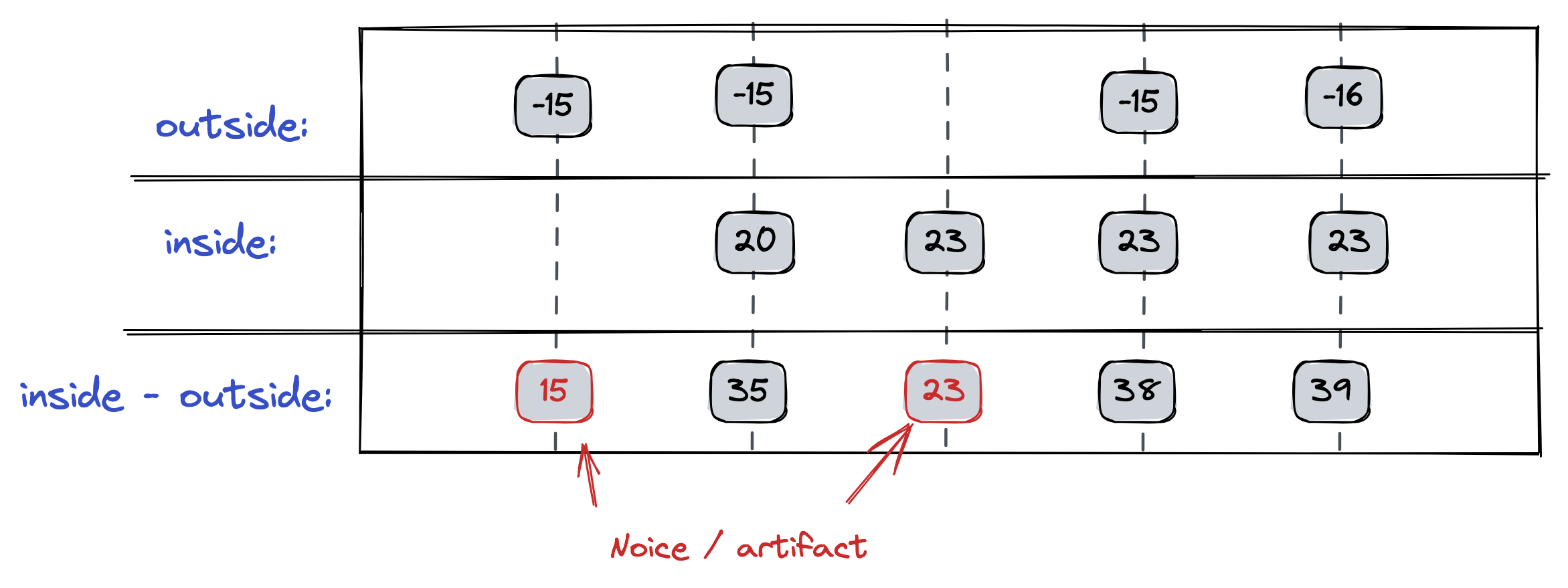# Changelog

New updates & improvements to Clarify.

## Calculated items:  Breaking change and new function

To improve the consistency in how operators work in calculated items, we are doing a breaking change to the result of  additions (+) and  subtractions (-)  when either of the input values contain empty values. To allow backwards compatibility for existing use-cases we have added a new function sum that retain the old behavior. The sum function is available now, while the changes to +/- will go live on Friday August the 25th 2023. This should give you some time to apply manual migrations, if you require it.

Is this change relevant to me?

If you use the + (or -) to summarize items that can be empty for any given calculation bucket, you should use the new sum function to retain the old behavior.

### Breaking change to +/- with empty values

We will change how + and - works when combining series that contain missing (empty) values. Before the breaking change goes live, we return a result for all time buckets where at least one of the input values contains a value. We do so by replacing the empty value with 0. This is different from how other operators work, such as * (multiplication) and / (division), which only return results when both inputs contain a value. After the change,  addition and subtraction will — just like all the other operators — only return a result when both inputs contain a value.

Example

To better understand the change, consider a calculation where you want to return the difference between the inside and the outside temperature, and consider what would happen if either of the series are missing a value. We have illustrated this below.Before the change we produce a result when at least one input contain a value. In this use-case, the result is not what we want.

### Introducing sum

Sometimes, you want to be able to summarize multiple series and return results, even for time buckets where one or more of the input values are empty. Before the breaking change, you will be able to do this using the + operator. However, if you want the calculation to work after the breaking change gets introduced, you must convert to using the sum function instead.

The sum function work by returning the sum of all non-empty inputs. I.e. sum(a, b, c) would work similar to how a + b + c worked before the breaking change.

### Migration old calculations

Before you migrate your existing calculations, you should consider which results you really want, rather than the results you got in the past. If you need help to understand what's best for you, please do not hesitate to contact Support.

Below is a short table of how to produce backwards compatible sums, that is sums that return results even when one of the inputs are empty.

Old syntax New syntax
a + b sum(a, b)
a + b + c sum(a, b, c)
a - b sum(a, -b)

If you are calculating the diff of two items, and where smart enough to figure out that you could use ternary conditions to omit empty values, you can simplify your expression once the breaking changes go live. Note that you are note required to do this simplification, as the old expression will continue to give valid results.

Old syntax New syntax
a != null && b != null ? a - b : null a - b

For more calculation options, check out our reference documentation.